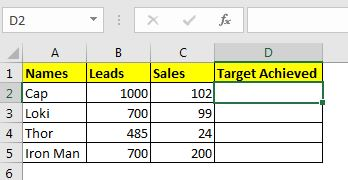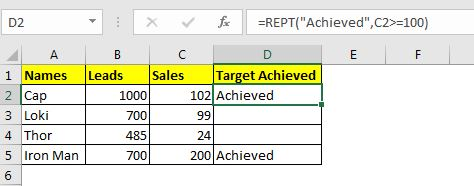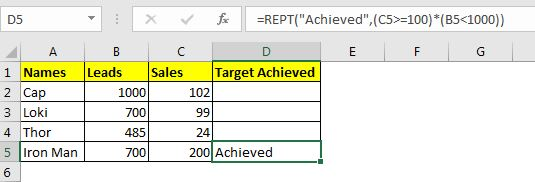# Return Conditional Output Using REPT Function

Yes, you read it right. The REPT function can be used to return conditional output. How? Let's see.

As we know the REPT function repeats the given expression to given times. We use this property as our advantage to get conditional output.

Syntax to get conditional output using REPT function:

 =REPT("Text",Condition)

Text: The message that you want to print when the condition is TRUE

Condition: The condition or the criteria that you want to check.

You may have got the Idea by now if you know about the REPT function and boolean values in excel. OK, let's have an example and see the formula in action.

### Example: Put "Achieved" if the target is met

Here, I have some sales records of powerful salesmen. The target of the sale is 100 sales. I want to put the text "Achieved" in the adjacent cell only if the salesman has done 100 or more sales.Write the below formula in cell D2 and copy down the cells.

 =REPT("Achieved",C2>=100)You can see that the formula puts "Achieved" in the respective cell only if the salesman has done 100 or more sales. Not impressed? You might think why not use IF function.

It can't even check multiple conditions. Wait, REPT function can check multiple conditions too.

Using REPT for Multiple Condition with AND logic

Let's say, in the above example, if you needed to put "Achieved" only if the salesman has done at least 100 sales and the Leads he had were less than 1000. In that case, the formula will be:

 =REPT("Achieved",(C2>=100)*(B2<1000))Maybe now you are impressed. The equivalent IF formula will be:

 =IF(C5>=100,IF(B5<1000,"Achieved",""),"")

Isn't the REPT function more elegant and fast? It is.

So, how does it work?

The method is simple. The condition is matched and if the condition is True (1) it returns the text message one time if the condition is False (0) then the REPT function returns the given text message zero times (means blank).

Let's see the second example. In the second example, we have 2 conditions to be met. The first is  (C2>=100)  which returns TRUE, the second criteria are (B2<1000) which returns FALSE. When we use multiple them we get the result as 0. Hence the formula returns the message zero times.

So yeah guys, this how you can use REPT function to get conditional output. I hope it was interesting. If you have any doubts regarding this topic or any other Excel/VBA related query, ask in the comments section below.

Related Articles:

How to use the REPT function in Excel | The Excel REPT function is a simple function, that prints a given text to given times. In this article, we will explore how REPT function works, how REPT functions can be used, and where REPT function is used best, what tricks can be done using this function

How to Find the Last ROW of data in Excel | To find the last row of data in an excel range we can use the REPT function and MATCH function together.

How to Remove leading and trailing spaces from text in Excel | The trailing spaces disturb your data set and it is necessary to remove any trailing or leading space from the text in excel. This formula removes all trailing spaces.

Popular Articles:

50 Excel Shortcuts to Increase Your Productivity | Get faster at your task. These 50 shortcuts will make you work even faster on Excel.

How to use Excel VLOOKUP Function| This is one of the most used and popular functions of excel that is used to lookup value from different ranges and sheets.

How to use the Excel COUNTIF Function| Count values with conditions using this amazing function. You don't need to filter your data to count specific value. Countif function is essential to prepare your dashboard.

How to Use SUMIF Function in Excel | This is another dashboard essential function. This helps you sum up values on specific conditions.

Terms and Conditions of use

The applications/code on this site are distributed as is and without warranties or liability. In no event shall the owner of the copyrights, or the authors of the applications/code be liable for any loss of profit, any problems or any damage resulting from the use or evaluation of the applications/code.